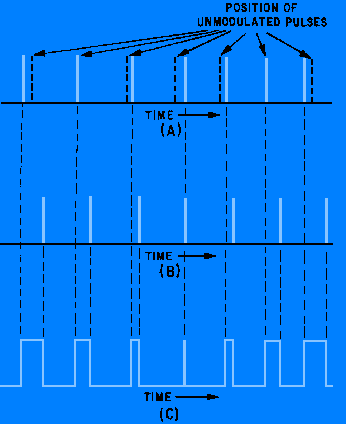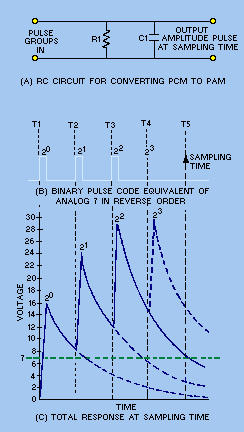waveform is averaged over both the pulse and no-pulse time, average voltage is the result. Since the amplitude of pdm pulses is constant, average voltage is directly proportional to pulse width. The pulse width varies with the modulation (intelligence) in pdm. Because the average value of the pulse train varies in accordance with the modulation, the intelligence may be extracted by passing the width-modulated pulses through a low-pass filter.">Custom SearchLOW-PASS FILTER Another method of demodulating pdm is by the use of a low-pass filter. If the voltage of a pulse waveform is averaged over both the pulse and no-pulse time, average voltage is the result. Since the amplitude of pdm pulses is constant, average voltage is directly proportional to pulse width. The pulse width varies with the modulation (intelligence) in pdm. Because the average value of the pulse train varies in accordance with the modulation, the intelligence may be extracted by passing the width-modulated pulses through a low-pass filter. The components of such a filter must be selected so that the filter passes only the desired modulation frequencies. As the varying-width pulses are applied to the low-pass filter, the average voltage across the filter will vary in the same way as the original modulating voltage. This varying voltage will closely approximate the original modulating voltage. CONVERSION Pulse-position modulation (ppm), pulse-frequency modulation (pfm), and pulse-code modulation (pcm) are most easily demodulated by first converting them to either pdm or pam. After conversion these pulses are demodulated using either peak detection or a low-pass filter. This conversion may be done in many ways, but your study will be limited to the simpler methods. Pulse-Position Modulation Ppm can be converted to pdm by using a flip-flop circuit. (Flip flops were discussed in NEETS, Module 9, Introduction to Wave-Generation and Wave-Shaping Circuits.) Figure 3-22 shows the waveforms for conversion of ppm to pdm. View (A) is the pulse-modulated pulse train and view (B) is a series of reset trigger pulses. The trigger pulses must be synchronized with the unmodulated position of the ppm pulses, but with a fixed time delay from these pulses. As the position-modulated pulse is applied to the flip-flop, the output is driven positive, as shown in view (C). After a period of time, the trigger pulse is again generated and drives the flip-flop output negative and the pulse ends. Because the ppm pulses are constantly varying in position with reference to the unmodulated pulses, the output of the flip-flop also varies in duration or width. This pdm signal can now be applied to one of the circuits that has already been discussed for demodulation. Figure 3-22. - Conversion of ppm to pdm.Pulse-frequency modulation is a variation of ppm and may be converted by the same method. Pulse-Code Modulation Pulse-code modulation can easily be decoded, provided the pulse-code groups have been transmitted in reverse order; that is, if the pulse with the lowest value is transmitted first, the pulse with the highest value is transmitted last. A circuit that will provide a constant value of current without regard to its load is known as a current source. A current source is used to apply the pcm pulses to an RC circuit, such as that shown in figure 3-23, view (A). The current source must be capable of supplying a linear charge to C1 that will increase each time a pulse is applied if C1 is not allowed to discharge between pulses. In other words, if C1 charges to 16 volts during the period of one pulse, then each additional pulse increases the charge by 16 volts. Thus, the cumulative value increases by 16 volts for each received pulse. This does not provide a usable output unless a resistor is chosen that allows C1 to discharge to one-half its value between pulses. If only one pulse is received at T1, C1 charges to 16 volts and then begins to discharge. At T2 the charge has decayed to 8 volts and continues to decay unless another pulse is received. At T3 it has a 4-volt charge and at T4 it only has a 2-volt charge. At the sampling time, a 1-volt charge remains; this charge corresponds to the binary-weighted pulse train of 0001. Now we will apply a pcm signal which corresponds to the binary-coded equivalent of 7 volts (0111) in figure 3-23, view (A). View (B) is the pulse code that is received. Remember that the pulses are transmitted in reverse order. View (C) is the response curve of the circuit. At T1 the pulse corresponding to the least significant digit is applied and C1 charges to 16 volts. C1 discharges between pulses until it reaches 8 volts at T2. At T2 another pulse charges it to 24 volts. At T3, C1 has discharged through R1 to a value of 12 volts. The pulse at T3 increases the charge on C1 by 16 volts to a total charge of 28 volts. At T4, C1 has discharged to one-half its value and is at 14 volts. No pulse is present at T4 so C1 will not receive an additional charge. C1 continues to discharge until T5 when it has reached 7 volts and is sampled to provide a pam pulse which can be peak detected. This sampled output corresponds to the original sampling of the analog voltage in the modulation. Figure 3-23. - Pcm conversion.When the pcm demodulator recognizes the presence or absence of pulses in each position, it reproduces the correct standard amplitude represented by the pulse code group. For this reason, noise introduces no error if the largest peaks of noise are not mistaken for pulses. The pcm signal can be retransmitted as many times as desired without the introduction of additional noise effects so long as the signal-to-noise ratio is maintained at a level where noise pulses are not mistaken for a signal pulse. This is not the only method for demodulating pcm, but it is one of the simplest. This completes your study of demodulation. You should remember that this module has been a basic introduction to the principles of modulation and demodulation. With the advent of solid-state electronics, integrated circuits have replaced discrete components. Although you cannot trace the signal flow through these circuits, the end result of the electronic action within the integrated circuit is the same as it would be with discrete components. Q.38 How does a low-pass filter detect pdm?Q.39 How is conversion used in pulse demodulation?Q.40 What is the discharge rate for the capacitor in a pcm converter?Integrated Publishing, Inc. - A (SDVOSB) Service Disabled Veteran Owned Small Business